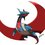# Proof of Von Mangoldt Dirichlet series

I am going to show a proof of $\sum_{n\geq 1}\dfrac{\Lambda(n)}{n^s}=-\dfrac{\zeta'(s)}{\zeta(s)}$ We start off by writing this as $\sum_{p=prime}\sum_{k=1}^\infty \dfrac{\ln(p)}{p^{sk}}=\sum_{p=prime} \dfrac{\ln(p)}{p^s-1}$ Let this be; we will use this later.

Lemma: $\sum_{n≥1} \dfrac{F(n)}{n^s}=\zeta(s)\sum_{p=prime}\dfrac{F(p)}{p^s-1}$ where F(n) is a completely additive function.

Proof: we can split the sum over primes. $F(p^k)=kF(p)$. we use this and get $\large\sum_{p=prime}\sum_{k=0}^\infty \dfrac{F(p^k)}{p^{sk}}\left(\sum_{p\not\mid n} \dfrac{1}{n^s}\right)=\sum_{p=prime}\sum_{k=0}^\infty \dfrac{kF(p)}{p^{sk}}\left(\zeta(s)-\zeta(s)p^{-s}\right)\\=\sum_{p=prime}F(p)\zeta(s)(1-p^{-s})\sum_{k=0}^\infty\dfrac{k}{p^{sk}}= \zeta(s)\sum_{p=prime}\dfrac{F(p)}{p^s-1}$

Now $\ln(n)$ is a completely additive function, so $\sum_{n=1}^\infty \dfrac{\ln(n)}{n^s}=\zeta(s)\sum_{p=prime}\dfrac{\ln(p)}{p^s-1}$ We know that $-\zeta'(s)=\sum_{n=1}^\infty \dfrac{\ln(n)}{n^s}$ and putting in the RHS's summation in terms of Von Mangoldt: $\sum_{n=1}^\infty \dfrac{\Lambda(n)}{n^s}=-\dfrac{\zeta'(s)}{\zeta(s)}$Note by Aareyan Manzoor
5 years, 5 months ago

This discussion board is a place to discuss our Daily Challenges and the math and science related to those challenges. Explanations are more than just a solution — they should explain the steps and thinking strategies that you used to obtain the solution. Comments should further the discussion of math and science.

When posting on Brilliant:

• Use the emojis to react to an explanation, whether you're congratulating a job well done , or just really confused .
• Ask specific questions about the challenge or the steps in somebody's explanation. Well-posed questions can add a lot to the discussion, but posting "I don't understand!" doesn't help anyone.
• Try to contribute something new to the discussion, whether it is an extension, generalization or other idea related to the challenge.

MarkdownAppears as
*italics* or _italics_ italics
**bold** or __bold__ bold
- bulleted- list
• bulleted
• list
1. numbered2. list
1. numbered
2. list
Note: you must add a full line of space before and after lists for them to show up correctly
paragraph 1paragraph 2

paragraph 1

paragraph 2

[example link](https://brilliant.org)example link
> This is a quote
This is a quote
    # I indented these lines
# 4 spaces, and now they show
# up as a code block.

print "hello world"
# I indented these lines
# 4 spaces, and now they show
# up as a code block.

print "hello world"
MathAppears as
Remember to wrap math in $$ ... $$ or $ ... $ to ensure proper formatting.
2 \times 3 $2 \times 3$
2^{34} $2^{34}$
a_{i-1} $a_{i-1}$
\frac{2}{3} $\frac{2}{3}$
\sqrt{2} $\sqrt{2}$
\sum_{i=1}^3 $\sum_{i=1}^3$
\sin \theta $\sin \theta$
\boxed{123} $\boxed{123}$

Sort by:

I have changed a typo: From "completely multiplicative function" to "completely additive function" (regarding ln(n))

Other than that, great proof!

Extra: Alternative proof of lemma:

$n=\prod _{p_j|n}^{ }p_j^{w_j}$

$F(n)=\sum _j^{ }w_jF\left(p_j\right)=\sum _{p^k|n}^{ }F\left(p\right)=1*f(n)P(n)$

Where f(n) satisfies f(p^k)=F(p) and P(n) is 1 if n=p^k and is 0 otherwise.

- 5 years, 4 months ago